CAT  >  CAT Quantitative Aptitude MCQ - 2

# CAT Quantitative Aptitude MCQ - 2

Test Description

## 34 Questions MCQ Test MBA Exams Mock Test Series and Past Year Question Papers | CAT Quantitative Aptitude MCQ - 2

CAT Quantitative Aptitude MCQ - 2 for CAT 2022 is part of MBA Exams Mock Test Series and Past Year Question Papers preparation. The CAT Quantitative Aptitude MCQ - 2 questions and answers have been prepared according to the CAT exam syllabus.The CAT Quantitative Aptitude MCQ - 2 MCQs are made for CAT 2022 Exam. Find important definitions, questions, notes, meanings, examples, exercises, MCQs and online tests for CAT Quantitative Aptitude MCQ - 2 below.
Solutions of CAT Quantitative Aptitude MCQ - 2 questions in English are available as part of our MBA Exams Mock Test Series and Past Year Question Papers for CAT & CAT Quantitative Aptitude MCQ - 2 solutions in Hindi for MBA Exams Mock Test Series and Past Year Question Papers course. Download more important topics, notes, lectures and mock test series for CAT Exam by signing up for free. Attempt CAT Quantitative Aptitude MCQ - 2 | 34 questions in 60 minutes | Mock test for CAT preparation | Free important questions MCQ to study MBA Exams Mock Test Series and Past Year Question Papers for CAT Exam | Download free PDF with solutions
 1 Crore+ students have signed up on EduRev. Have you?
CAT Quantitative Aptitude MCQ - 2 - Question 1

### 5 men and 6 women are to be arranged in a straight line for a photograph. In how many ways this can be done such that two particular women Sheela and Chameli are always together and no two men are beside each other?

Detailed Solution for CAT Quantitative Aptitude MCQ - 2 - Question 1

Since two women are always together, 6 women can be arranged in 5! x 2! = 240 ways.
Now, 5 men can stand at 6 different gaps created between the women.
They can be arranged in 6P5 = 6! = 720 ways

So, total number of ways = 240 x 720 = 172800

Hence, option 2.

CAT Quantitative Aptitude MCQ - 2 - Question 2

### What is the highest power of 48 in 32!?

Detailed Solution for CAT Quantitative Aptitude MCQ - 2 - Question 2

48= 24 *3

Highest power of 3 in 32! = 32/3+10/3+3/3

=10+3+1

= 14

Highest power of 2 in 32! = 32/2+16/2+8/2+4/2+2/2

= 16+8+4+2+1

=31

Highest power of  24 in 32!= 7

*Answer can only contain numeric values
CAT Quantitative Aptitude MCQ - 2 - Question 3

### What will be the number of zeroes at the end of the product of the first 40 natural numbers?

Detailed Solution for CAT Quantitative Aptitude MCQ - 2 - Question 3

Number of zeroes in a number can be found by calculating the highest power of 10.

10 = 2 x 5

Number of zeroes will be highest power of 5 in 40!.
Highest power of 5 in 40!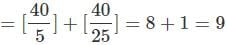CAT Quantitative Aptitude MCQ - 2 - Question 4

The speed of the boat in still water is 6 km/h and the speed of the river is 1.2 km/h. Boat takes a total of 10 h to go to a place and come back. What is the total distance covered in the whole process?

Detailed Solution for CAT Quantitative Aptitude MCQ - 2 - Question 4

Let us assume D is the distance.
Upstream Speed = 4.8 km/h
Downstream speed = 7.2 km/h
According to the question, D/4.8 + D/7.2 = 10
So, D = 28.8 km and
Hence the total distance = 57.6 km
Alternatively, the ratio of downstream speed: upstream speed = 3:2
Ratio of the downstream time: upstream time = 2:3 The time taken in the downstream movement = 4 h and the time taken in the upstream movement = 6 h
So, the distance covered = 4 x 7.2 = 6 x 4.8 = 28.8 km
Hence the total distance = 57.6 km
In the case of escalators, moving staircase works like an external agent as the river works for boats and streams. The speed of an escalator and the person will be added when the staircase is going up and the person walking up with it have the same direction of the movement. Now if the direction of the movement of an escalator and the person is opposite, then the resultant speed (or, the relative speed) will be equal to the speed of the person — to the speed of an escalator.

CAT Quantitative Aptitude MCQ - 2 - Question 5

The total number of relations from set P to set Q are 4096. If x and y are the number of elements in sets P and Q respectively, find the number of values the ordered pair (x, y) can take.

Detailed Solution for CAT Quantitative Aptitude MCQ - 2 - Question 5

Let us define a function from a set P of 3 elements (a, b, c) and a set Q with 4 elements (w, x, y, z). Now, for a function to be defined from set P to set Q, every element of P must connect with exactly one element of Q while every element of Q can connect to multiple elements of P.
Thus, the three elements of set P each has four options from Q. Thus, the total number of relations in this case = 43 = 64.
Now, the number of relations given is 4096. xy = 4096

(x, y) = (4096, 1); (2, 12); (4, 6); (8, 4); (16, 3); (64, 2)

Hence, there are 6 such pairs possible.
Hence, option 4.

*Answer can only contain numeric values
CAT Quantitative Aptitude MCQ - 2 - Question 6

The annual income of X and Y is in the ratio 6 : 5 and their expenses are in the ratio 9 : 7. If each saves Rs. 3000 at the end of the year, find the sum of their annual incomes.

Detailed Solution for CAT Quantitative Aptitude MCQ - 2 - Question 6

Let the income of X and Y be 6x and 5x respectively.
Let he expenses of X and Y be 9y and ly respectively.

6x- 9y = 3000 ... (1)

5x- 7y = 3000 ... (2)

By solving these two equations we get,

42x - 45x = -6000

x = 2000

Therefore, income of x = 2000 x 6 = 12000 And income of Y = 2000 x 5 = 10000

Sum of their incomes = 12000 + 10000 = 22000

CAT Quantitative Aptitude MCQ - 2 - Question 7

The sum of three numbers is zero and the the product of the three numbers is -120, find the sum of the cubes of the three numbers.

Detailed Solution for CAT Quantitative Aptitude MCQ - 2 - Question 7

If a + b + c = 0, a* + 63 + c3 = 3 abc

The sum of the cubes of three numbers = -120 x 3 = -360

Hence, option 3.

CAT Quantitative Aptitude MCQ - 2 - Question 8

The per month average salary of the employees of a firm is Rs. 20,000. All the employees are given a raise of 15% after the appraisal month. However, some people resigned from the firm and the funds allocated towards giving away their salary was distributed amongst all. Resultantly, the average rise in the salary of the currently working employees increased by Rs. 2,000. If 46 employees are currently working with the company then find the number of employees who resigned.

Detailed Solution for CAT Quantitative Aptitude MCQ - 2 - Question 8

Let the number of employees who left be x.
The total funds allocated towards the salary after increment = (46 + x) x 20000 x 1.15

The new salary per employee = 20000 x 1.15 + 2000 = 25000

(46 + x) x 20000 x 1.15 = 25000 x 46 Solving for x, we get

x = 4

Hence, option 1.

CAT Quantitative Aptitude MCQ - 2 - Question 9

The 3rd term of an A.R is 23 and 8th term is 58. Find the 80th term.

Detailed Solution for CAT Quantitative Aptitude MCQ - 2 - Question 9

Let the 1st term (t1) of an A.R be a and common difference be d.

t3 = a + 2d = 23 ... (i)

Similarly,

t8 = a + 7d = 58 ... (ii)

Solving these equation we get,

a = 9 and d = 7

t8= a + 79d = 9 + 79(7) = 562

Hence, option 2.

CAT Quantitative Aptitude MCQ - 2 - Question 10

On planet K-Pax, the dial of a clock has 14 equally spaced markings. The day is divided into 28 hours; each hour is divided into 70 minutes and each minute is divided into 70 seconds. Find that number of times that the hour hand and minute are exactly opposite to each other in a day.

Detailed Solution for CAT Quantitative Aptitude MCQ - 2 - Question 10

Consider the clock at 14 AM in the night.

The hour hand and minute hand are opposite to each other once before the time is 1 AM. They are again opposite to each other once before the time is 2 AM. And so on in every hour till 7 AM.

Between 6 AM and 8 AM, the hands are opposite to each other only once, at 7 AM. Again the hands are opposite to each other once every hour from 9 AM to 14 noon.

So, in a span of 14 hours, the number of times that the two hands are opposite to each other is 13.

So, in 28 hours, the number of times that the two hands are opposite to each other is 13*2 = 26

CAT Quantitative Aptitude MCQ - 2 - Question 11

What is the value of x + √x, if log2 X + log2 X2 + log2 X3 +...+ log2 X10= 440?

Detailed Solution for CAT Quantitative Aptitude MCQ - 2 - Question 11

The equation can be written as, (1 + 2 + 3 + ...+ 10)log2x = 440
(11 x 10/2)log2x = 440
log2X = 8
x = 28 = 256
Thus, x = 256 and √x = 16
∴ x + √x = 272
Hence, option 3.

CAT Quantitative Aptitude MCQ - 2 - Question 12

A train 125 m long passes a man, running at 5 km/hr in the same direction in which the train is going, in 10 seconds. The speed of the train is:

Detailed Solution for CAT Quantitative Aptitude MCQ - 2 - Question 12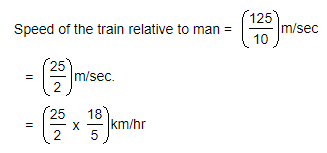= 45 km/hr.

Let the speed of the train be x km/hr. Then, relative speed = (x - 5) km/hr.x - 5 = 45x = 50 km/hr.

CAT Quantitative Aptitude MCQ - 2 - Question 13

The ratio of the sum to product of two digits of a two-digit number is 2 : 3. If one of the digits exceeds the other by 4, then find the number of highest value which can be formed using the two digits.

Detailed Solution for CAT Quantitative Aptitude MCQ - 2 - Question 13

Let one of the digits be x.
∴ The other digit will be x + 4
Now,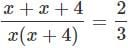6x + 12 = 2x2 + 8x
x2 + x - 6 = 0
x = 2, -3
Since, -3 cannot be one of the digits the digits are 2 and 6.
Thus, 62 is the number with highest value which can be formed using the two digits.
Hence, option 2.

CAT Quantitative Aptitude MCQ - 2 - Question 14

What will be the co-ordinates of the foot of the perpendicular drawn from the point (3, 4) to the line 4x + 2y - 9 = 0?

Detailed Solution for CAT Quantitative Aptitude MCQ - 2 - Question 14

Let (a, b) is the required co-ordinates of the foot of the perpendicular.

4a + 2b - 9 = 0 ... (i)

Slope of the above line = -2

Slope of the line perpendicular to the above line =1/2 (b - 4)/(a - 3) = 1/2

a-2b = —5 ... (ii)

Solving the equations (i) and (ii) we get,

a = 4/5 and b = 29/10

Hence, option 2.

CAT Quantitative Aptitude MCQ - 2 - Question 15

The roots of the quadratic equation x2 + bx + c = 0, having distinct roots, are non-zero and integral solutions of the inequality, y2 + 2y - 3 < 0. What is the value of b + c?

Detailed Solution for CAT Quantitative Aptitude MCQ - 2 - Question 15

y2 + 2y - 3 = (y - l)(y + 3) < 0

-3 < y < 1

y can take the integral values -2, -1, 0

Roots o f the given equation can be - 2 , - 1 , 0

As roots are non-zero, therefore, the roots of the equation x2 + bx + c = 0 are -2,-1.
x2 + bx + c = (x + 2)(x + 1) = 0

x2 + bx + c = x2 + 3x + 2 = 0

b = 3 and c = 2

b + c = 5

Hence, option 2.

*Answer can only contain numeric values
CAT Quantitative Aptitude MCQ - 2 - Question 16

A family spends Rs. 3,000 per month to purchase 15 kg grocery. If the rate per kg of the grocery increases by 20%, how much less grocery (in kg) should the family purchase to keep the expenditure same?

Detailed Solution for CAT Quantitative Aptitude MCQ - 2 - Question 16

Original per kg cost of grocery = 3000/15 = Rs. 200

New rate = 1.2 x 200 = Rs. 240

Grocery purchased after increase in price = 3000/240 =12.5kg

Reduction in consumption = 15 - 12.5 = 2.5 kg

CAT Quantitative Aptitude MCQ - 2 - Question 17

What is the diagonal of a square (in cm) having its area equal to that of a rhombus having its diagonals as 12 cm and 14 cm?

Detailed Solution for CAT Quantitative Aptitude MCQ - 2 - Question 17

Area of rhombus = (1/2) x 12 x 14 = 84 cm2 Let the diagonal of the square be d

(1/2) x d2 = 84

Diagonal of the square = d = 2√42 cm Hence, option 1.

*Answer can only contain numeric values
CAT Quantitative Aptitude MCQ - 2 - Question 18

A train 440 metres long passes a railway platform 876 metres long in 10 seconds. How many seconds will it take to pass a running train 990 metres long? (Enter 0 if the answer cannot be determined.)

Detailed Solution for CAT Quantitative Aptitude MCQ - 2 - Question 18

As we do not know in which direction the other train is running, we cannot determine answer.

CAT Quantitative Aptitude MCQ - 2 - Question 19

Find the selling price of a sari after successive discounts of 20%, 25% and 30% on the marked price of Rs. 700.

Detailed Solution for CAT Quantitative Aptitude MCQ - 2 - Question 19

Selling price after successive discount = 700 x 0.8 x 0.75 x 0.7 = Rs. 294

Hence, option 1.

CAT Quantitative Aptitude MCQ - 2 - Question 20

Two circles with different radii touch each other in one point such that the smaller circle lies completely inside the larger circle. The smaller circle has point O as the center. A chord AB of the larger circle is tangent to the smaller circle. AB touches smaller circle at point C. A and B lies on the circumference of the larger circle. If BC = 10 cm and OB = 20 cm. Find OC (in cm).

Detailed Solution for CAT Quantitative Aptitude MCQ - 2 - Question 20

AB touches smaller circle at C. So, OC ⊥ AB.
Now, in AOCB, OB = 20 cm and BC =10 cm. By pythagoras theorem we get, OC = 10√3 cm
Hence, option 1.

*Answer can only contain numeric values
CAT Quantitative Aptitude MCQ - 2 - Question 21

The average salary per employee in an office is Rs. 3,200 per month. The average salary of officers is Rs. 6,800 and that of non-officers is Rs. 2,000. If the number of officers is 5, then find the number of non-officers in the office.

Detailed Solution for CAT Quantitative Aptitude MCQ - 2 - Question 21

Let the number of officers and non-officers be n and m respectively.

Total salary of officers = 6800n And, Total salary of non-officers = 2000m

Total salary of all the employees = 6800n + 2000m = 3200(m + n)

3600n = 1200m

3n = m

m = 3 x 5 = 15

Number of non-officers =15

CAT Quantitative Aptitude MCQ - 2 - Question 22

In a set of 17 points, exactly 5 points are collinear. How many triangles can be formed using these points? (Assume all points are distinct)

Detailed Solution for CAT Quantitative Aptitude MCQ - 2 - Question 22

We need to select exactly three points from the given 17 points to form a triangle.
However, no triangle can be formed if all the three points selected are collinear.
Number o f triangles formed = 17C3 - 5C3 = 670

Hence, option 4.

CAT Quantitative Aptitude MCQ - 2 - Question 23

The perimeter of an isosceles triangle is equal to 14 cm and the ratio of the lateral side and the base is 5 : 4. Find the area of the triangle (in cm2)

Detailed Solution for CAT Quantitative Aptitude MCQ - 2 - Question 23

Let 5x be the lateral side of the triangle and 4x be the base of the triangle.
5x + 5x + 4x= 14
x =1
On solving the two equation we get y = 4 and x = 5.
We get the following triangle.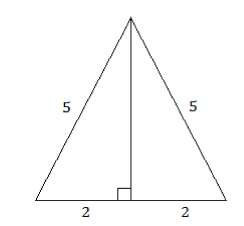By Pythagoras theorem,
Height of the triangle = √52 - 22 = √21 cm
Hence, area of the triangle = 1/2 x 4 x √21 = 2√21 cm2
Hence, option 4.

CAT Quantitative Aptitude MCQ - 2 - Question 24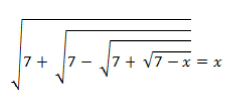Which of the following can be value of x?

Detailed Solution for CAT Quantitative Aptitude MCQ - 2 - Question 24

On substituting the values from the options given above, we see that x = 3 is the only value that satisfies the given equation.
Hence, option 2.

*Answer can only contain numeric values
CAT Quantitative Aptitude MCQ - 2 - Question 25

If 'f' is the real function satisfying 2f(xy) = [f(x)]y + [f(y)]x for all real values of x, y and f(1) = 2, then f(1) +f(2)+f(3) + ... +f(10) is equal to

Detailed Solution for CAT Quantitative Aptitude MCQ - 2 - Question 25

2f[xy) = [f(x)]y + [f(y)]x for all real x, y Replacing ‘y’ by 1, we get,
∴ 2 x f(x) = [f(x)]+ [f(1)]x
∴ f(x) = 2x
∴ f(2) = 22 = 4,f(3) = 23 = 8 and so on.
∴ f(1) +f(2) + ... + f(10) = 21 + 22 + 23 + ... + 210
The above series is in G.R with ratio = 2 and initial term = 2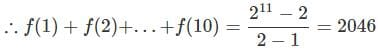CAT Quantitative Aptitude MCQ - 2 - Question 26

If 343x+2 - 49x+3 + 7x+4 = 0, which of these could be a value for x?

Detailed Solution for CAT Quantitative Aptitude MCQ - 2 - Question 26

343x+2 - 49x+3 + 7x+4 = 73x + 6 - 72x+6 + 7x+4
∴ 7x+4(72x +2 - 7x+2 + 1) = 0
Now, 7x+4 ≠ 10
∴ 72x + 2 - 7x+2 + 1 = 0
Let 7x+1 = y
∴ y - 7y + 1 = 0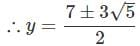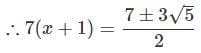Taking log7 on both sides,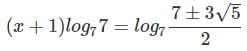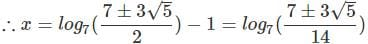Hence, option 3.

CAT Quantitative Aptitude MCQ - 2 - Question 27

The length of the common tangent of two circles of different radius is 15 cm and it divides the line joining the centre of the two circles in the ratio 2 : 1. Find the area of the larger circle if the length of the line joining the centre of two circles is 39 cm.

Detailed Solution for CAT Quantitative Aptitude MCQ - 2 - Question 27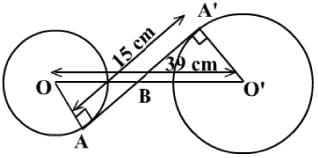Consider the given diagram.
∠ OBA ≌ ∠O'BA'... (Vertically opposite angles)
∠ OAB ≌ Z.O'A'B ... (Radius is perpendicular to the tangent)
∴ ΔOAB ~ AO'A'B ... (AA test of similarity)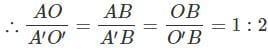Given that OO ' = 39 cm and AA' = 15 cm

O'B = 26 cm and A'B = 10 cm

O'A' = (O'B2 - A'B2)1/2 = 24 cm

Area of the larger circle = 576π

Hence, option 2.

CAT Quantitative Aptitude MCQ - 2 - Question 28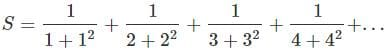What is the value of S?

Detailed Solution for CAT Quantitative Aptitude MCQ - 2 - Question 28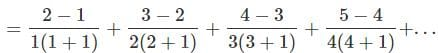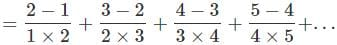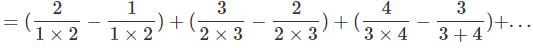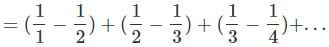= 1
Hence, option 3.

*Answer can only contain numeric values
CAT Quantitative Aptitude MCQ - 2 - Question 29

Sushil is standing 25 m away from a tower. If the angle of elevation of the tower from this point is 45°, find the height (in m) of the tower.

Detailed Solution for CAT Quantitative Aptitude MCQ - 2 - Question 29

The angle of elevation is 45°.
So, height of the tower will be same as the distance of the tower from Sushil.
The height of the tower is 25 m

CAT Quantitative Aptitude MCQ - 2 - Question 30

X alone takes 5 days and Y alone takes 7 days to finish a job. How many days will X and Y take to finish the job working on alternate days starting with X?

Detailed Solution for CAT Quantitative Aptitude MCQ - 2 - Question 30

Let the total work be LCM of number of days taken by X and Y individually.

Total work = LCM (5, 7) = 35 unit

In 2 days, X & Y will complete (12/35)th of the total work.
In this manner they will complete (24/35)th work in 4 days.
After this X will work for 1 day and he completes 1/5th work.
After 5th day, work left = (11/35 - 1/5) = 4/35 On 6th day, Y will do the remaining 4/35th work in 4/5th of days time.
So total time taken is 5 + 4/5 days.
Hence, option 4.

CAT Quantitative Aptitude MCQ - 2 - Question 31

In a geometric progression, there is exactly one term between the terms 24 and 3456. What will be the ratio of the 4th term to the 2nd term of the geometric progression?

Detailed Solution for CAT Quantitative Aptitude MCQ - 2 - Question 31

Let r be the common ratio of the geometric progression.
The fourth term of the geometric progression, t4 =t2 x r2, where t2 is the second term of the geometric progression.

t:t2 = r:1

Also, 3456 = 24 x r2 => r2 = 144

t4 : t2 = 144 : 1

Hence, option 4.

CAT Quantitative Aptitude MCQ - 2 - Question 32

Some quantity of juice from a container of 60 litres of apple juice is replaced with mango juice. Same quantity of the mixture is drawn again and is replaced by equal quantity of mango juice. After this, quantity of apple juice left in the container is (4/9)th of the initial quantity. What quantity of the liquid is taken out from the container both time (in litres)?

Detailed Solution for CAT Quantitative Aptitude MCQ - 2 - Question 32

Let the qunatity drawn from the container both times be x.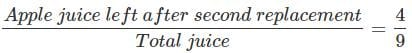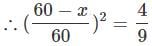Solving this we get, x = 20 litres
Hence, option 2.

*Answer can only contain numeric values
CAT Quantitative Aptitude MCQ - 2 - Question 33

What is the number of real roots of the equation px2 + qx| + r = 0, where p, q and r are positive real numbers?

Detailed Solution for CAT Quantitative Aptitude MCQ - 2 - Question 33

px2 + q|x|+ r = 0

p , q and r are positive, x2 and x are also non-negative. The sum o f nonnegative and positive numbers cannot be zero.

The equation has no real roots.

*Answer can only contain numeric values
CAT Quantitative Aptitude MCQ - 2 - Question 34

In the fig., ST = 6 cm, QR = 10 cm and PS = 9 cm. Find PR (in cm).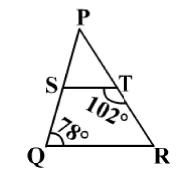Detailed Solution for CAT Quantitative Aptitude MCQ - 2 - Question 34

In the ΔPQR and ΔPTS,

m∠QPR = m∠TPS ... (Same angles with different names)

m∠PTS + m∠STR = 180°

m∠PTS = 180° - m∠STR = 180° - 102° = 78°

Also, m∠PQR = 78°

m∠PQR = m∠PTS

ΔPQR ~ ΔPTS
PR / PS = QR / TS
PR = QR x PS / TS = 10 x 9 / 6 = 15

## MBA Exams Mock Test Series and Past Year Question Papers

59 tests
 Use Code STAYHOME200 and get INR 200 additional OFF Use Coupon Code
Information about CAT Quantitative Aptitude MCQ - 2 Page
In this test you can find the Exam questions for CAT Quantitative Aptitude MCQ - 2 solved & explained in the simplest way possible. Besides giving Questions and answers for CAT Quantitative Aptitude MCQ - 2, EduRev gives you an ample number of Online tests for practice

## MBA Exams Mock Test Series and Past Year Question Papers

59 tests

### How to Prepare for CAT

Read our guide to prepare for CAT which is created by Toppers & the best Teachers